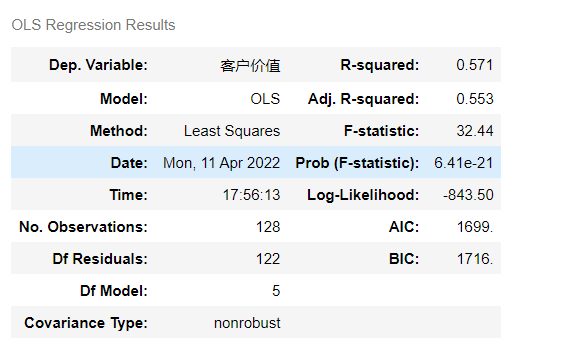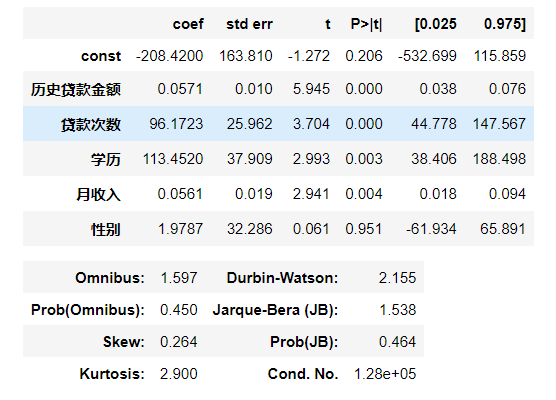# 1 多元线性回归简介# 2 案例：客户价值预测模型

## 2.2 具体代码

``````import pandas as pd
from sklearn.linear_model import LinearRegression

X = df[['历史贷款金额','贷款次数','学历','月收入','性别']]
Y = df['客户价值']
model = LinearRegression()
model.fit(X,Y)

model.coef_,model.intercept_``````

y=-208+0.057x^1+96x^2+113x^3+0.056x^4+1.98x^5

# 3 模型评估

``````import statsmodels.api as sm
est = sm.OLS(Y,X2).fit()
est.summary()``````# 4 线性回归优缺点

·优点：快速；没有调节参数；可轻易解释；可理解。

·缺点：相比其他复杂一些的模型，其预测准确率不高，因为它假设特征和响应之间存在确定的线性关系，这种假设对于非线性的关系，线性回归模型显然不能很好地进行数据建模。

# 参考书籍

《Python大数据分析与机器学习商业案例实战》